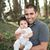# Alok Menghrajani

Previously: security engineer at Square, co-author of HackLang, put the 's' in https at Facebook. Maker of CTFs.

Ajsone is an esoteric programming language designed for fun. The language abuses JSON by using the JSON data format to represent everything: functions, variables, values, etc.

If you have never played with esoteric languages, keep in mind that writing even the most trivial algorithms can be mindblowing ().

Before looking at how the language works, here is a simple piece of code which computes the factorial of 5:

```{
"fact": {
"=if": {
"cond": {"=<=": {"in1": "=n", "in2": 1}},
"then": 1,
"else": {
"t": {"=-": {"in1": "=n", "in2": 1}},
"=*": {"in1": "=n", "in2": {"=fact": {"n": "=t"}}}}
}
},
"=fact": {"n": 5}
}
```

Ajsone represents everything as JSON. Given that everyone knows JSON, there is no need to go into the details of the grammar.

Tying the grammar to JSON has some disadvantages. Serious users are therefore encouraged to implement a pre-processor to support features like comments or trailing commas.

Ajsone is a functional (as in functional programming, not to be confused with useful), untyped , lazy, and dynamically scoped programming language.

I initially tried making the language lazy evaluation based, but I wasn't able to get things to work in a "reasonable" way. I kept running into weird recursive definitions due to dynamic scope.

Most JSON values have their usual semantics. Strings which begin with the '=' character and JSON objects get special treatment.

• "hello world" -> the "hello world" string.
• 12.34 -> a number between 12 and 13.
• ["foo", "bar", 42] -> an array with 3 elements
• true -> a boolean
• false -> the other boolean
• null -> null

JSON key-value objects represent functions.

JSON key-value objects which contain other key-value objects as values are nested functions.

The last key of a key-value object is the return value of the function. E.g. the following code assigns `1` to `x` and returns the value of `x`, i.e. returns `1`:

```{
"x": 1
}
```

Each function creates a new dynamic scope, which continues to live in the nested functions. In the following piece of code, `x` is assigned `1`, and `y` is just a temporary variable.

There is no explicit variable declaration, so the scope starts when a variable is first assigned.

```{
"x": { "y": 1 }
}
```

Strings which begin with `=` are evaluated (think of it as function application or variable de-reference depending of the context).

The following piece of code assigns `1` to `x` and then assigns the value of `x` to `y`.

```{
"x": 1,
"y": "=x"
}
```

And is equivalent to:

```{
"x": 1,
"y": {"=x": null}
}
```

Note: since JSON keys must be unique within an object, Ajsone code feels like static single assignment (SSA).

There is no "main", the JSON tree represents the entire program, and the last expression is the result of the program.

The following program reads the declaration of `x` and `y` and returns the value of `z`. `z` overwrites `x` and returns the result of `y`, which ends up being 2.

The following program returns 2.

```{
"x": 1,
"y": { "foo": "=x"},
"z": {
"x": 2,
"_": {"bar": "=y"}
}
}
```

Functions don't declare their inputs, they just read variable from the global scope. This imples parameters can be omitted. The following two pieces of code are (almost) equivalent:

```{
"arr": [1, 2, 3],
"=arr.len": { "arr": "=arr" }
}
```
and:
```{
"arr": [1, 2, 3],
"=arr.len": {}
}
```

When using recusion, we need a way to create local variables. The way this is achieved is by pushing the value part of the key-value function call on a new scope and discarding it when the function returns.

The language provides the following built-in operators. The argument types for each of these built-ins is checked at runtime.

• if: checks `cond` and returns `=then` when true, `=else` otherwise.

• +, -, *, /: usual math operators. Numerical parameters are expected in `in1` and `in2`.
• <, <=, >, >=, ==, !=: usual comparator operators. Numerical parameters are expected in `in1` and `in2`.
• &&, ||: usual boolean operators. Boolean parameters are expected in `in1` and `in2`.
• &, |, ~: usual binary operators. Numerical parameters are expected in `in1` and `in2`.

• str.len: returns the length of string `s`.
• str.at: returns the `offset`th element of string `s`.
• str.append: returns a new strings, which is the result of appending string `s1` to string `s2`.

• arr.len: returns the length of array `arr`.
• arr.at: returns the `offset`th element of array `arr`.
• arr.append: returns a new array, which is the result of appending `e` to array `arr`.
• arr.prepend: returns a new array, which is the result of prepending `e` to array `arr`.

Feel free to try out Ajsone, using the following interpreter. You can try running the following pieces of code: